## Example Questions

### Example Question #1 : How To Find The Angle Of Clock Hands

What is the angle between the hour and minute hand of a clock at 4:15?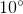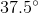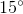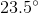Explanation:

In this case, the minute hand is pointing at 3 while the hour hand is pointing at some value past 4. Now, since 15 minutes represents 1/4 of an hour, the hour hand will be 1/4 the distance between 4 and 5. The total number of degrees between minutes is 360/12 = 30°; therefore, the hour hand is 30/4 or 7.5° past the 4 o'clock point. Now, between 3 and 4, there are 30°.  Add to that the 7.5°.  The total distance is therefore 37.5°.

### Example Question #1 : How To Find The Angle Of Clock Hands

What is the angle, in degrees, between the minute and hour hands of the clock at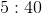pm?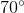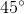Explanation:

To find the degrees between hands on a clock, you must first remember that a clockface is a circle, and therefore has an internal angle sum of 360 degrees. Since a clockface has a sum of 360 degrees and there are 12 numbers on a clockface, the degree difference between each number is 360 divided by 12, or 30 degrees.

You can use this 30 degrees to figure out the distance between the minute and hour hands at the time given.  At 5:40pm, the minute hand will be on the 8, as each number indicates five minutes on the clock.

It is the hour hand where this calculation gets tricky. While it would be easy to assume that the hour hand would be on the 5 at this time, that's actually incorrect.  The hour hand slowly creeps towards the next number throughout the hour, moving in appropriate increments to reflect the fraction of the hour that has past.  Since 40 minutes is 2/3 of an hour, then the hour hand is 2/3 of the way towards the number 6 on 5:40pm.

Thus, when calculating the distance, you must find the difference between 5 2/3 and 8 on the clock.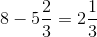Multiply this number by 30 (the degrees between each number on the clock, and thus the way we must convert this number to degrees), and you get 70 degrees.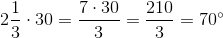### Example Question #6 : Clock Math

A clock has two equally long hands on it, each measuringinches. If the minute hand is directly onand the hour hand is directly on, what is the distance between the two hands?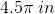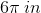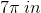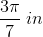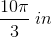Explanation:

Our clock looks roughly like this: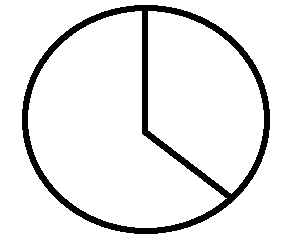Now, between every number on the clock, there are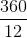ordegrees. Therefore, fromto, there are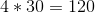degrees. To find the arc length, you use the equation: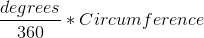Now, we know:We know that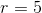. Therefore, we can write our equation: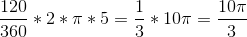### Example Question #8 : Clock Math

A clock has two equally long hands on it, each measuringinches. If the minute hand is directly onand the hour hand is directly in the middle ofand, what is the distance between the two hands?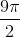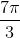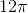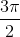Explanation:

Our clock looks roughly like this: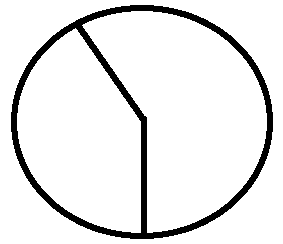Now, between every number on the clock, there areordegrees. We have a little trickier math to do, however. Let's subdivide the clock intosubsections instead. Each of these will havedegrees. Now, betweenand, there aresuch subsections. Since the hour hand is directly in the middle ofand, there is one more such subsection. Therefore, we havetotal subsections or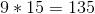degrees. To find the arc length, you use the equation:Now, we know:We know that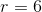.  Therefore, we can write our equation: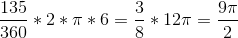### Example Question #7 : Clock Math

A clock has two equally long hands on it, each measuringinches and extending to the edge of the clock. If the minute hand is directly onand the hour hand is directly on, what is the length of the minor arc connecting the two hands?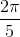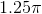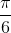Explanation:

Our clock looks roughly like this: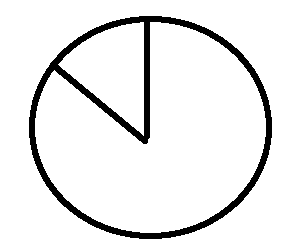Now, between every number on the clock, there areordegrees. Therefore, fromtothere areof these sectors. Therefore, there are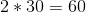degrees. To find the arc length, you use the equation:Now, we know:We know that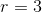. Therefore, we can write our equation: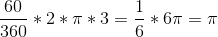Tired of practice problems?

Try live online GRE prep today.